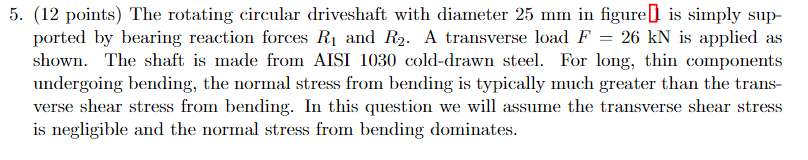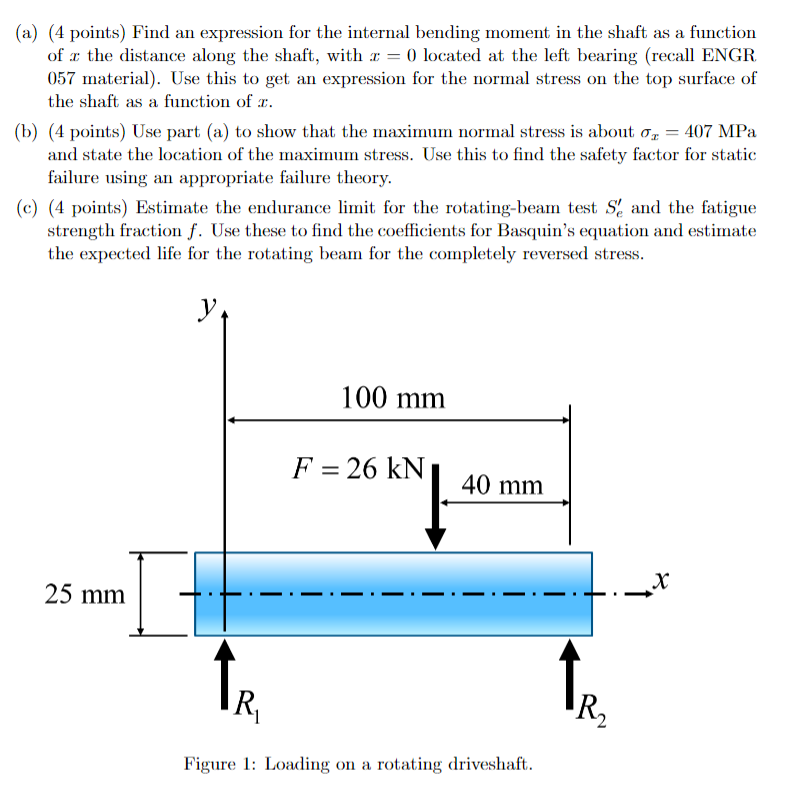# Question 5. (12 points) The rotating circular driveshaft with diameter $$25 \mathrm{~mm}$$ in figure [ is simply supported by bearing reaction forces $$R_{1}$$ and $$R_{2}$$. A transverse load $$F=26 \mathrm{kN}$$ is applied as shown. The shaft is made from AISI 1030 cold-drawn steel. For long, thin components undergoing bending, the normal stress from bending is typically much greater than the transverse shear stress from bending. In this question we will assume the transverse shear stress is negligible and the normal stress from bending dominates. (a) (4 points) Find an expression for the internal bending moment in the shaft as a function of $$x$$ the distance along the shaft, with $$x=0$$ located at the left bearing (recall ENGR 057 material). Use this to get an expression for the normal stress on the top surface of the shaft as a function of $$x$$. (b) (4 points) Use part (a) to show that the maximum normal stress is about $$\sigma_{x}=407 \mathrm{MPa}$$ and state the location of the maximum stress. Use this to find the safety factor for static failure using an appropriate failure theory. (c) (4 points) Estimate the endurance limit for the rotating-beam test $$S_{e}^{\prime}$$ and the fatigue strength fraction $$f$$. Use these to find the coefficients for Basquin's equation and estimate the expected life for the rotating beam for the completely reversed stress. Figure 1: Loading on a rotating driveshaft.AMQWGO The Asker · Mechanical EngineeringTranscribed Image Text: 5. (12 points) The rotating circular driveshaft with diameter $$25 \mathrm{~mm}$$ in figure [ is simply supported by bearing reaction forces $$R_{1}$$ and $$R_{2}$$. A transverse load $$F=26 \mathrm{kN}$$ is applied as shown. The shaft is made from AISI 1030 cold-drawn steel. For long, thin components undergoing bending, the normal stress from bending is typically much greater than the transverse shear stress from bending. In this question we will assume the transverse shear stress is negligible and the normal stress from bending dominates. (a) (4 points) Find an expression for the internal bending moment in the shaft as a function of $$x$$ the distance along the shaft, with $$x=0$$ located at the left bearing (recall ENGR 057 material). Use this to get an expression for the normal stress on the top surface of the shaft as a function of $$x$$. (b) (4 points) Use part (a) to show that the maximum normal stress is about $$\sigma_{x}=407 \mathrm{MPa}$$ and state the location of the maximum stress. Use this to find the safety factor for static failure using an appropriate failure theory. (c) (4 points) Estimate the endurance limit for the rotating-beam test $$S_{e}^{\prime}$$ and the fatigue strength fraction $$f$$. Use these to find the coefficients for Basquin's equation and estimate the expected life for the rotating beam for the completely reversed stress. Figure 1: Loading on a rotating driveshaft.
More
Transcribed Image Text: 5. (12 points) The rotating circular driveshaft with diameter $$25 \mathrm{~mm}$$ in figure [ is simply supported by bearing reaction forces $$R_{1}$$ and $$R_{2}$$. A transverse load $$F=26 \mathrm{kN}$$ is applied as shown. The shaft is made from AISI 1030 cold-drawn steel. For long, thin components undergoing bending, the normal stress from bending is typically much greater than the transverse shear stress from bending. In this question we will assume the transverse shear stress is negligible and the normal stress from bending dominates. (a) (4 points) Find an expression for the internal bending moment in the shaft as a function of $$x$$ the distance along the shaft, with $$x=0$$ located at the left bearing (recall ENGR 057 material). Use this to get an expression for the normal stress on the top surface of the shaft as a function of $$x$$. (b) (4 points) Use part (a) to show that the maximum normal stress is about $$\sigma_{x}=407 \mathrm{MPa}$$ and state the location of the maximum stress. Use this to find the safety factor for static failure using an appropriate failure theory. (c) (4 points) Estimate the endurance limit for the rotating-beam test $$S_{e}^{\prime}$$ and the fatigue strength fraction $$f$$. Use these to find the coefficients for Basquin's equation and estimate the expected life for the rotating beam for the completely reversed stress. Figure 1: Loading on a rotating driveshaft.&#12304;General guidance&#12305;The answer provided below has been developed in a clear step by step manner.Step1/4(a) To find the internal bending moment in the shaft as a function of x, we can use the formula for the bending moment in a simply supported beam with a point load at the center: $$\mathrm{{M}{\left({x}\right)}={F}\times{\left(\frac{{L}}{{2}}-{X}\right)}}$$where F is the transverse load, L is the length of the beam, and x is the distance along the beam. In this case, $$\mathrm{{L}={100}{m}{m}}$$ and x is measured from the left bearing, so we have:$$\mathrm{{M}{\left({x}\right)}={26}\times{\left({50}-{X}\right)}}$$To find the normal stress on the top surface of the shaft as a function of a, we can use the formula for the normal stress due to bending: $$\mathrm{\sigma_{{x}}={M}\times\frac{{c}}{{I}}}$$where c is the distance from the neutral axis to the top surface of the shaft, and I is the second moment of area. For a circular shaft with diameter d, we have: $$\mathrm{{c}=\frac{{d}}{{2}}}$$ $$\mathrm{{I}=\frac{{\pi\times{d}^{{4}}}}{{64}}}$$In this case,$$\mathrm{{d}={25}{m}{m}}$$ , so we have:$$\mathrm{{c}={12.5}{m}{m}}$$$$\mathrm{{I}=\frac{{\pi\times{25}^{{4}}}}{{64}}={122{,}934}{m}{m}^{{4}}}$$ Therefore, the normal stress on the top surface of the shaft as a function of x is:$$\mathrm{\sigma_{{x}}{\left({x}\right)}={M}{\left({x}\right)}\frac{{c}}{{I}}=\frac{{{26}{\left({50}-{x}\right)}{12.5}}}{{\frac{{\pi{25}^{{4}}}}{{64}}}}={131.7}\times{\left({50}-{x}\right)}}$$ ExplanationPlease refer the solution in this step.Explanation:Please refer to solution in this step.Step2/4(b) The maximum normal stress occurs at the location where $$\mathrm{\sigma_{{x}}}$$is a maximum. To find this, we can take the derivative of $$\mathrm{\sigma_{{x}}}$$with respect to x and set it equal to zero:$$\mathrm{\frac{{{d}\sigma_{{x}}}}{{{\left.{d}{x}\right.}}}=-{131.7}={0}}$$This gives $$\mathrm{{x}={50}{m}{m}}$$ as the location of the maximum stress. To find the maximum stress, we can substitute $$\mathrm{{x}={50}{m}{m}}$$ into the expression for $$\mathrm{\sigma_{{x}}}$$: $$\mathrm{\sigma_{{x}}{\left(\max\right)}={131.7}\times{\left({50}-{50}\right)}={0}{M}{P}{a}}$$However, this is the stress on the neutral axis, which is not the location of maximum stress. To find the maximum stress, we need to add the normal stress due to bending on the bottom surface of the shaft, which is equal in magnitude to the stress on the top surface but opposite in sign. Therefore, the maximum normal stress is:$$\mathrm{\sigma_{{x}}={2}\times\sigma_{{x}}{\left(\max\right)}={0}{M}{P}{a}}$$This is below the yield stress of \( \mathrm{{A}{I}{S}{I}{1},{030}} \ ... See the full answer